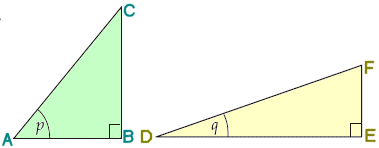Go to content
These formulae are often refered to as the addition formulae:
\sin(A \pm B) = \sin A \cos B \pm \cos A \sin B
\cos(A \pm B) = \cos A \cos B \mp \sin A \sin B
\tan(A \pm B) = \displaystyle \frac{\tan A \pm \tan B}{1 \mp \tan A \tan B}You can get a better display of the maths by downloading special TeX fonts from jsMath. In the meantime, we will do the best we can with the fonts you have, but it may not be pretty and some equations may not be rendered correctly.

Glossary

the trigonometrical formulae:
sin(A+B)=sinAcosB+cosAsinB
sin(A-B)=sinAcosB-cosAsinB
cos(A+B)=cosAcosB-sinAsinB
cos(A-B)=cosAcosB+sinAsinB

union

The union of two sets A and B is the set containing all the elements of A and B.

Full Glossary List

This question appears in the following syllabi:

SyllabusModuleSectionTopicExam Year
AQA A-Level (UK - Pre-2017)C4TrigonometryAddition formulae-
AQA A2 Maths 2017Pure MathsFurther TrigonometryAddition Formulae-
AQA AS/A2 Maths 2017Pure MathsFurther TrigonometryAddition Formulae-
CBSE XI (India)Sets and FunctionsTrigonometric FunctionsAddition identities e.g. sinx +siny sin(x+y) etc.-
Edexcel A-Level (UK - Pre-2017)C3TrigonometryAddition formulae-
Edexcel A2 Maths 2017Pure MathsTrigonometric Problem SolvingAddition Formulae-
Edexcel AS/A2 Maths 2017Pure MathsTrigonometric Problem SolvingAddition Formulae-